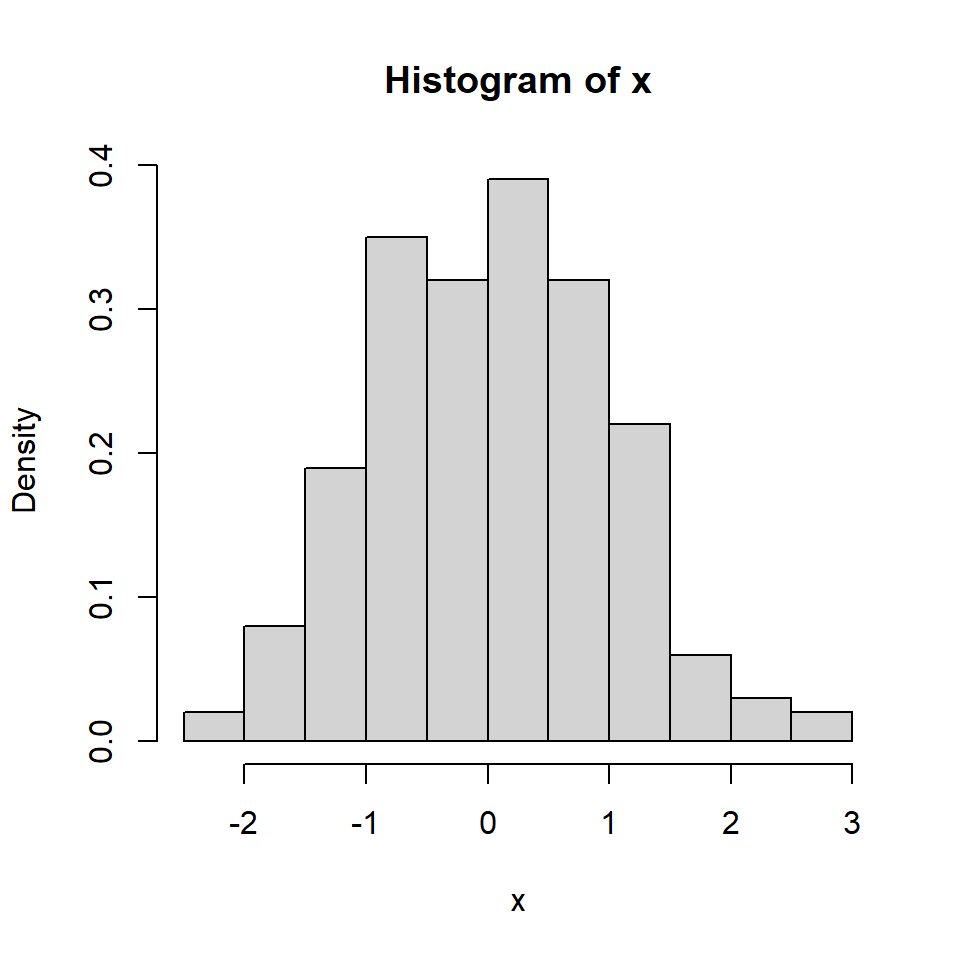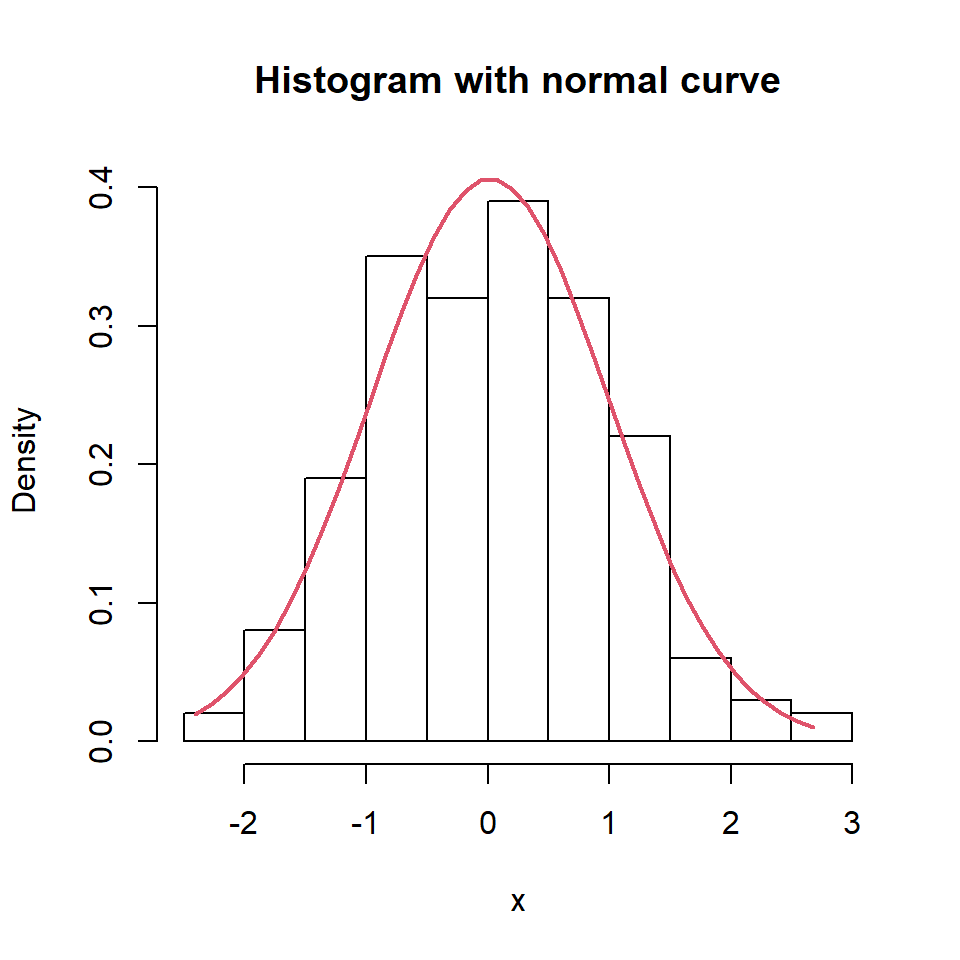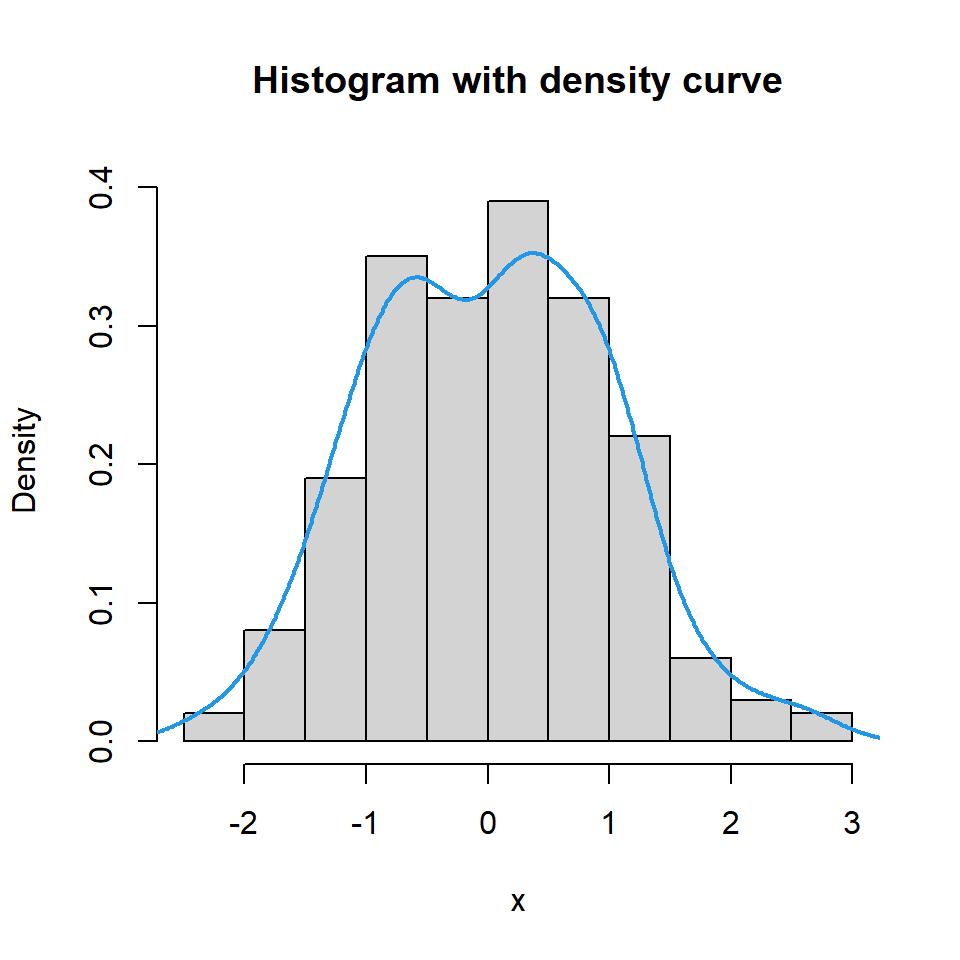# Histogram with density curves in R

A basic histogram can be created with the `hist` function. In order to add a normal curve or the density line you will need to create a density histogram setting `prob = TRUE` as argument.

``````# Sample data
set.seed(3)
x <- rnorm(200)

# Histogram
hist(x, prob = TRUE)``````## Histogram with normal curveIf you want to overlay a normal curve over your histogram you will need to calculate it with the `dnorm` function based on a grid of values and the mean and standard deviation of the data. Then you can add it with `lines`.

``````# X-axis grid
x2 <- seq(min(x), max(x), length = 40)

# Normal curve
fun <- dnorm(x2, mean = mean(x), sd = sd(x))

# Histogram
hist(x, prob = TRUE, col = "white",
ylim = c(0, max(fun)),
main = "Histogram with normal curve")
lines(x2, fun, col = 2, lwd = 2)``````

## Histogram with density line

If you prefer adding the density curve of the data you can make use of the `density` function as shown in the example below.

``````# Sample data
set.seed(3)
x <- rnorm(200)

# Histogram
hist(x, prob = TRUE, ylim = c(0, max(fun)),
main = "Histogram with density curve")
lines(density(x), col = 4, lwd = 2)``````